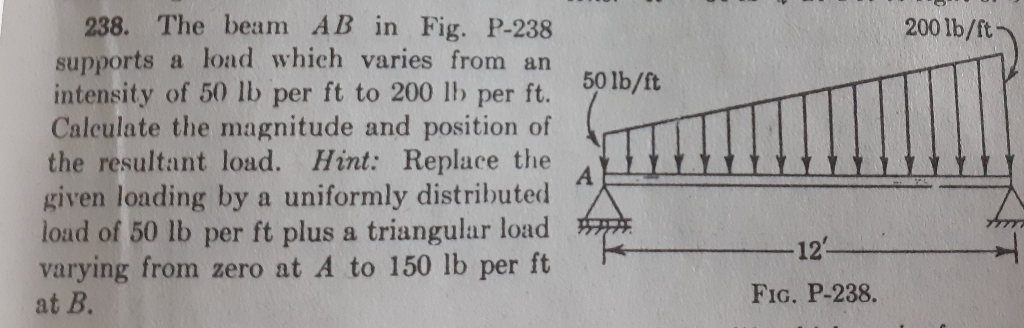# Question 200 lb/ft 50 lb/ft 238. The beam AB in Fig. P-238 supports a load which varies from an intensity of 50 lb per ft to 200 lb per ft. Calculate the magnitude and position of the resultant load. Hint: Replace the given loading by a uniformly distributed load of 50 lb per ft plus a triangular load varying from zero at A to 150 lb per ft at B. -121 Fig. P-238.YTNRJC The Asker · Other MathematicsTranscribed Image Text: 200 lb/ft 50 lb/ft 238. The beam AB in Fig. P-238 supports a load which varies from an intensity of 50 lb per ft to 200 lb per ft. Calculate the magnitude and position of the resultant load. Hint: Replace the given loading by a uniformly distributed load of 50 lb per ft plus a triangular load varying from zero at A to 150 lb per ft at B. -121 Fig. P-238.
More
Transcribed Image Text: 200 lb/ft 50 lb/ft 238. The beam AB in Fig. P-238 supports a load which varies from an intensity of 50 lb per ft to 200 lb per ft. Calculate the magnitude and position of the resultant load. Hint: Replace the given loading by a uniformly distributed load of 50 lb per ft plus a triangular load varying from zero at A to 150 lb per ft at B. -121 Fig. P-238.